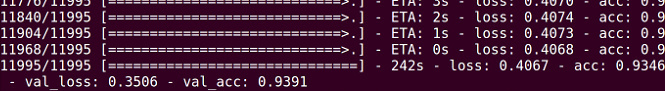# Manual training/validation set accuracy calculations

I’m trying to deploy my trained model, i wanted first to make sure that the preprocessing step that i’m making after reading the image through opencv and swapping channels … etc is working well.

After training my model vgg16bn with batch-normalization , here’s the final outputAnd here’s the model definition

def create(self, size, include_top):
if size != (224,224):
include_top=False

``````    model = self.model = Sequential()
``````
``````    self.ConvBlock(2, 64)
self.ConvBlock(2, 128)
self.ConvBlock(3, 256)
self.ConvBlock(3, 512)
self.ConvBlock(3, 512)
``````
``````    if not include_top:
fname = 'vgg16_bn_conv.h5'
return
``````
``````    model.add(Flatten())
self.FCBlock()
self.FCBlock()
``````
``````    fname = 'vgg16_bn.h5'
``````

After the training i save the model and the weights, then i reload them in production and start classifying with the following code

srcfile = root + ‘/’ + file
#print srcfile
img = cv2.imread(root + ‘/’ + file)
img = cv2.resize(img,(224, 224)).astype(np.float32)
img[:,:,0] -= 103.939 #b channel
img[:,:,1] -= 116.779 #g channel
img[:,:,2] -= 123.68 #r channel
img = img.transpose((2,0,1))
img = np.expand_dims(img,axis= 0)
preds , n , m= vgg.predict(img)

and m contains the classification output
I start counting the right classifications and divide by the number of images in the training set and evaluation set, expecting to have the same output as reported in the training

But the reported accuracy on the training set is: 94.08%
and on the validation set is: 92.968

Is that due to the shuffle option in the fit process? or am i doing something wrong with my data? is the way i’m using to measure accuracy wrong?
Another question, why do i have to subtract the mean from the image before predicting while the model contains as a first step the vgg_preprocess function

def vgg_preprocess(x):

``````    vgg_mean = np.array([123.68, 116.779, 103.939], dtype=np.float32).reshape((3,1,1))
x = x - vgg_mean
return x[:, ::-1] # reverse axis rgb->bgr
``````

Thank you so much in advance